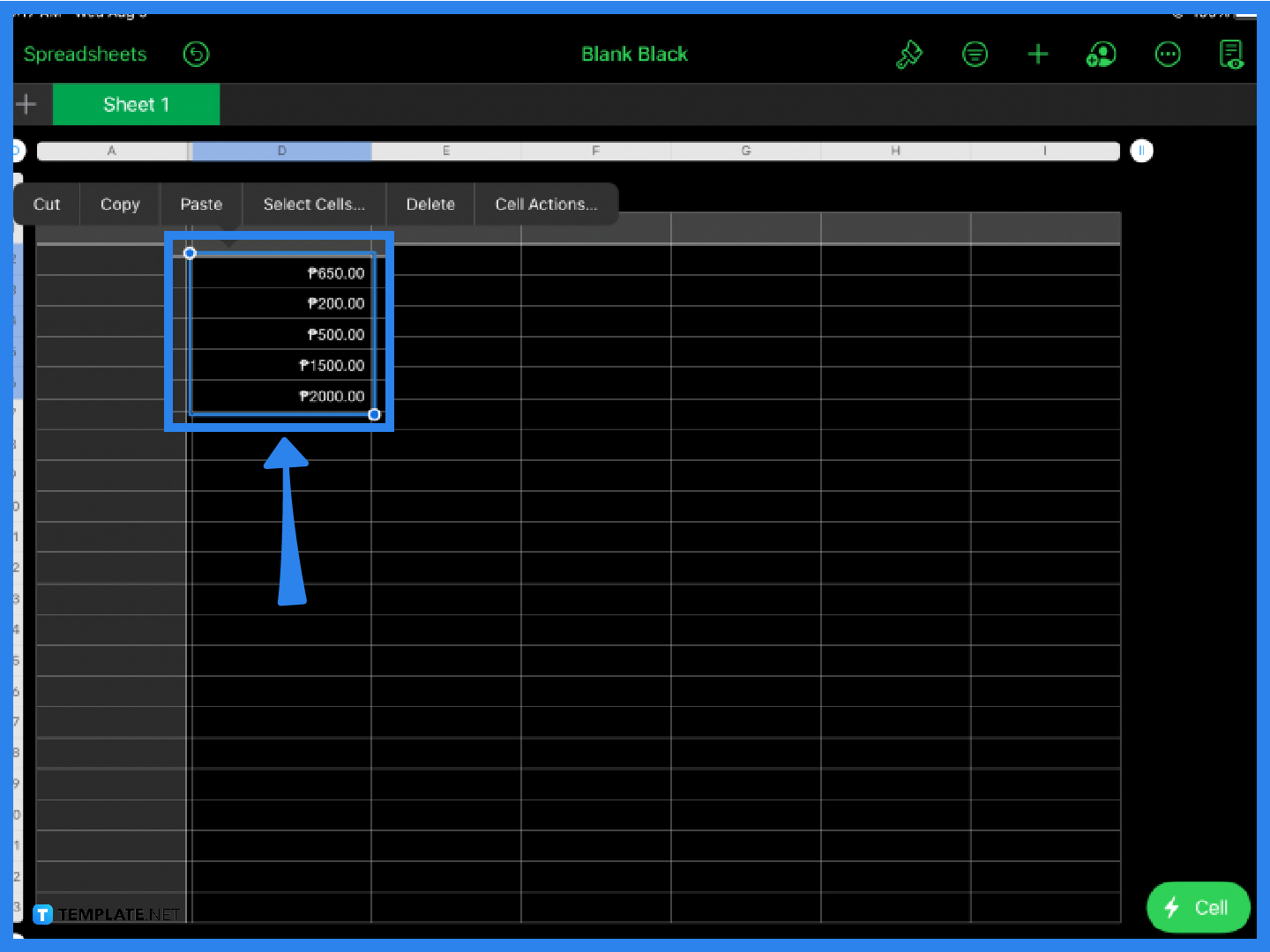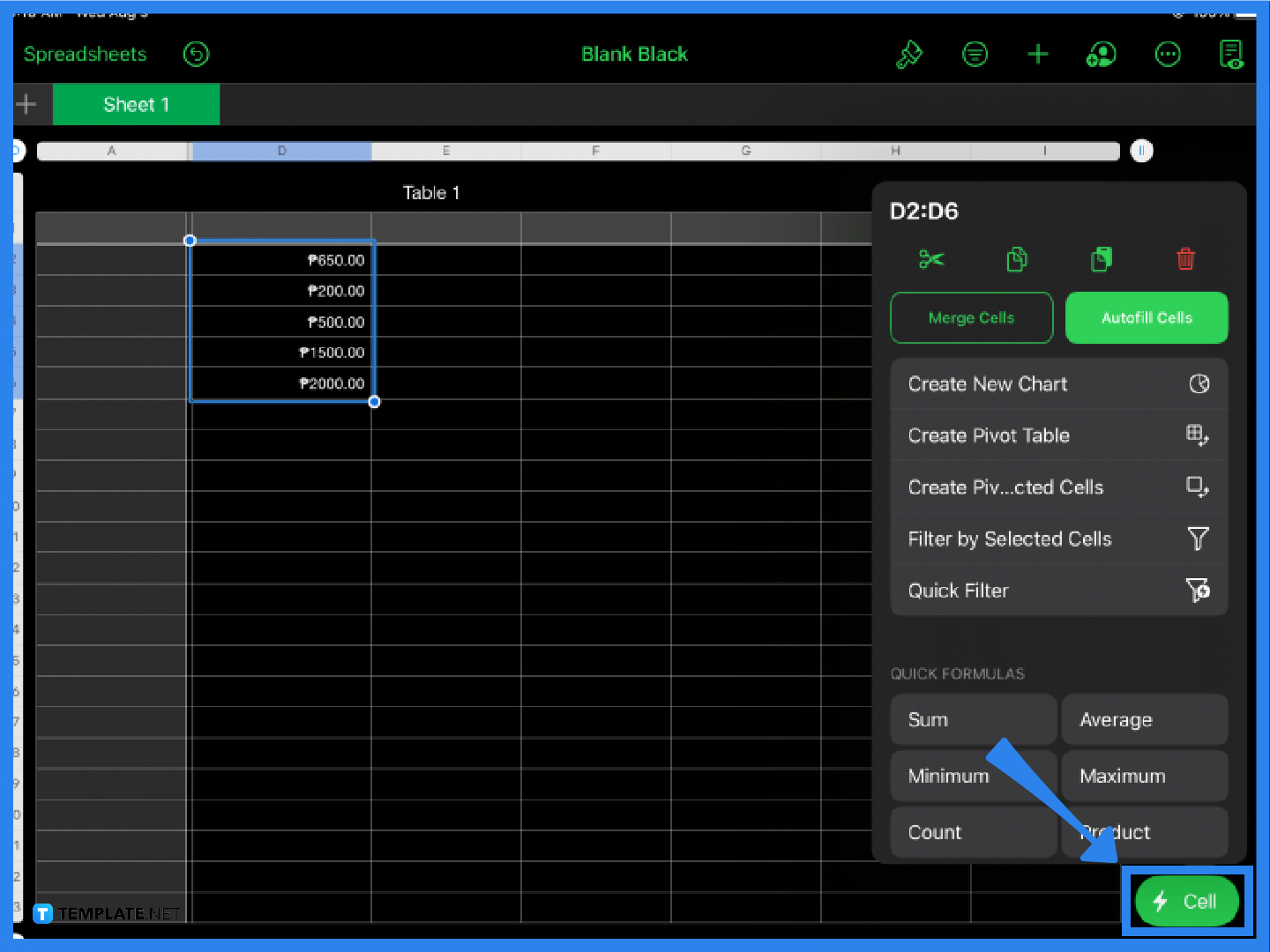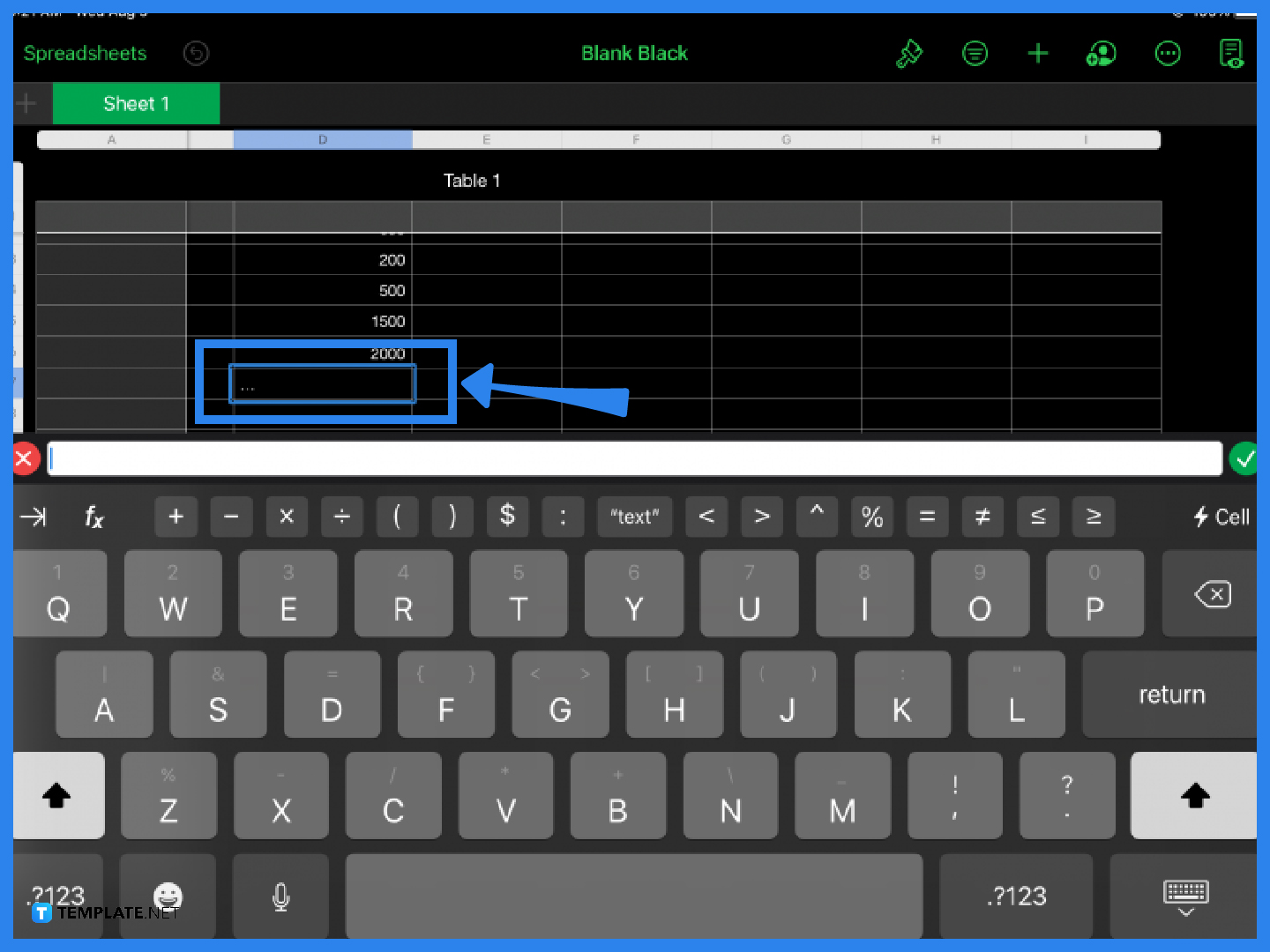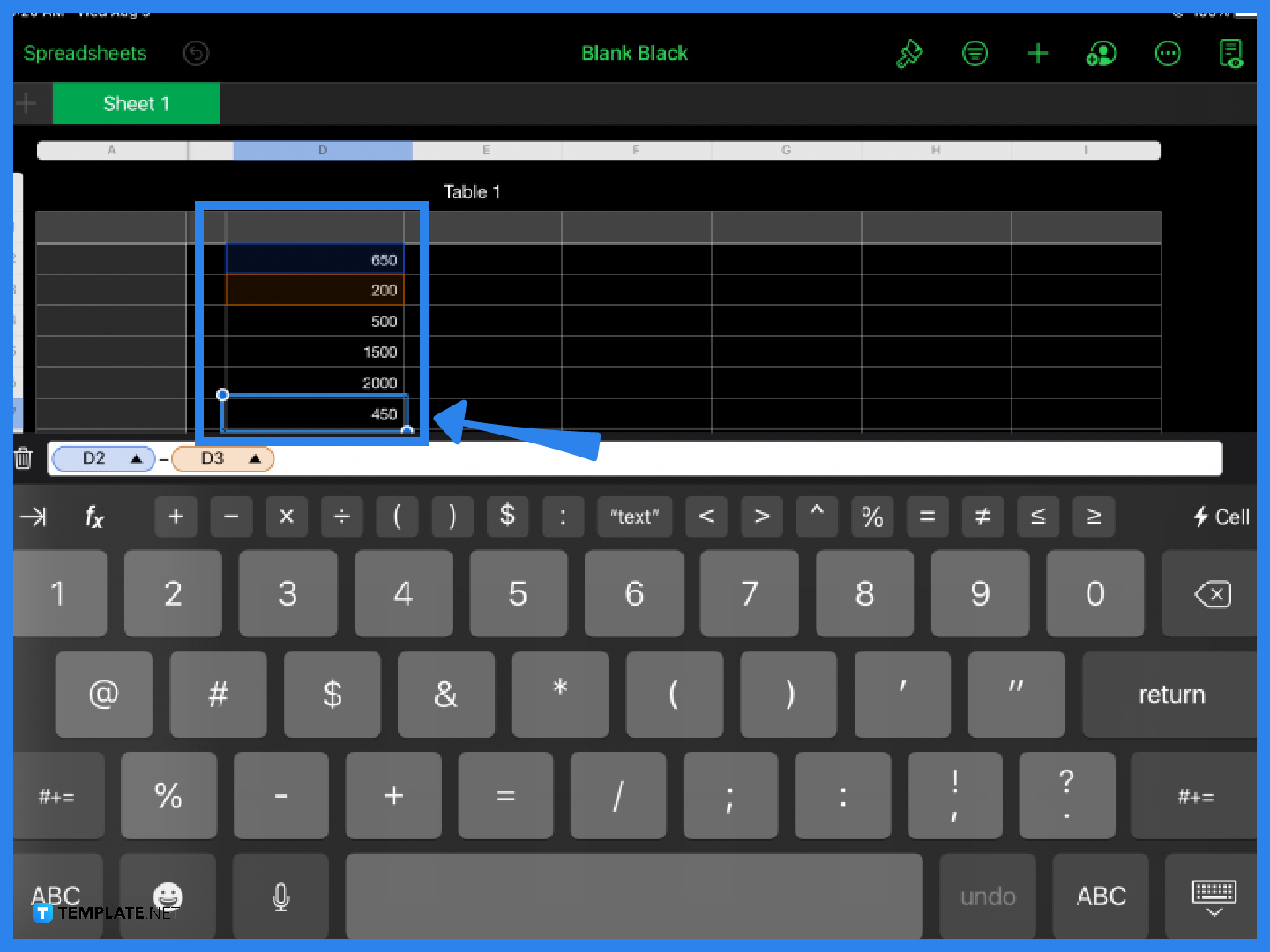# How to Add or Subtract in Apple Numbers

Addition and subtraction of numbers are not just basic functions in Apple Numbers; they are fundamental concepts in Mathematics. As soon as a young child or student can learn how to count, the next step is usually learning the basics of adding and subtracting numbers.## How to Add or Subtract in Apple Numbers

In Apple Numbers, you do not have to manually add or subtract values and numbers. Follow these simple steps and learn how to use Apple Numbers’ addition and subtraction functions.

• ### Step 1: Input the DataYou cannot use the add or subtract function in Apple Pages with a blank cell or spreadsheet. The first step is to input your values or numbers. These may be expenses, quotas, quantities, or whatever your specific spreadsheet requirements are.

• ### Step 2: Click the Cell IconOnce you have listed and arranged your values, select all or specific ones that you want to add. Click on the Cell icon on the lower right side of the screen and under Quick Formulas, select Sum. With Apple Numbers, you can also perform other key formulas such as finding the average, minimum, and product of your spreadsheet values.

• ### Step 3: Use the Formula BarIf you prefer to see the formula bar instead, click on the cell below the last value or any cell and type in the equal (=) sign. This will instantly display the formula bar. The formula bar is where you can find mathematical functions, including addition and subtraction functions.

• ### Step 4: Change the FunctionTo get the sum or the difference of two or more values, simply type in the cell name or location then choose either the addition (+) or subtraction (-) function from the formula bar. Hit Enter or click the green check mark on the rightmost side of the formula bar to get the sum or difference. The answer will automatically appear in your spreadsheet.

## FAQs

### How do you add in Apple Numbers?

A fast and simple way to add values in Apple Numbers is by selecting the values you want to be added, clicking the Cell function, and choosing Sum from the Quick Formulas menu.

### How do you subtract two cells in Apple Numbers?

Click on any cell, type in the = sign, key in the cell names (e.g., B4, D8) to be subtracted, choose the minus (-) sign on the formula bar, and hit Enter or click the green check to get the result.

### How do you create a subtraction formula in Apple Numbers?

To perform simple subtraction in Apple Numbers, click any cell in your spreadsheet, type the equal sign, the first value, followed by the minus sign, and the second value, then hit Enter.

### What is minus in Apple numbers?

Apple Numbers does not have an explicit function for subtraction; however, you can get the difference of values or numbers by using the formula bar and the minus sign (-) instead.

### Is Apple numbers as good as Excel?

Both Apple Numbers and Microsoft Excel have similar functions, but the question of whether or not one is better than the other is largely a matter of individual preferences and needs.